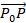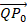-->

# Vector Line and Field Equations in 3rd Dimension

In this article, the notion of vectors is used to reduce the equation of lines and fields in space-3, which is very useful in other mathematical fields.

### Equation Field in Space-3

A field in space-3 can be determined by giving its inclination and by specifying one of its points. The method that makes it easy to explain the inclination is to assign a vector (called normal) that is perpendicular to the field.

Suppose we want the equation of field passing through point P0(X0, Y0, Z0) and has a nonzero vector n = (a, b, c) as normal. Based on Figure 1, it is clear that the field consists of points P (x, y, z) where the vectoris orthogonal to n, i.e.

We will call this the normal form of the field equation.
By multiplying it and by gathering its terms the equation of the field can be rewritten in the form.

ax + by + cz + d = 0

where a, b, c and d are constants which are not all zero.

Example 1.
Find the equation of the field that passes point (3, -1, 7) and perpendicular to vector n = (4, 2, -5).
Solution:
By using the normal form point,

Then we get,
4(x - 3) + 2(y - 1) + 5(z - 7)=0
or
4x + 2y – 5z + 25 = 0

### Line Equations in dimension 3

Let l be a line in space-3 through point P0 (x0, y0, z0) and parallel to the nonzero vector v = (a, b, c).

It is clear that Figure 2, I exactly consists of points p(x, y, z) for which vectorsare parallel with v, ie there is a scalar t so,

The components of the equation above can we write as,

We get that equation

x = x0 + ta
y = y0 + tb  where -∞ < t < + ∞
Z = z0 + tc
These equations are called line parameter equations.

Example 2.
Lines through points (1, 2, -3) and parallel to vectors v = (4, 5, -7) have a parameter equation

X = 1 + 4a
Y = 2 + 5b  where -∞ < t < + ∞
Z = -1 - 7c

Example 3.
Find the parameter equation for the intersection of fields.
3x + 2y – 4z -6 = 0                           and                             x – 3y – 2z- 4 = 0

Solution:
The intersection line consists of all points (x, y, z) that fulfill both equations in the system

3x + 2y – 4z -6 = 0
x – 3y – 2z- 4 = 0

By completing both equations this will produce the parameter equation for that line

Because there are an infinite number of fields passing through that line, there are always infinitely many pairs of fields like that. To find two such fields if a, b, and c are not equal to zero, we can write each equation.

x = x0 + ta
y = y0 + tb                  where -<t<+
z = z0 + tc

in the form of

By eliminating the parameter t will show that the line consists of all points (x, y, z) that satisfy the equation.

which is called the symmetric equation for that line. So, the line can be seen as the intersection of fields

or as the intersection of

Example 4.
Find the equation of two fields whose intersections are lines

x = 3 + 2t
y = -4 + 7t                  where -<t<+
z = 1 + 3t

Solution:
Because the symmetric equation for this line is

Then this line is the intersection of fields

Or equivalent,

7x – 2y – 29 = 0       and    3y – 7z + 19 = 0

If we can find the distance between a point and a field, we can find the distance between fields parallel by calculating the distance between one field and point P0 in another field.

Theorem 1.
The distance D between points P0(x0, y0, z0) with the field ax + by + cz + d = 0 is
Proof:
Suppose that Q (x1, y1, z1) is any point in that field. Normal position n = (a, b, c) so that the starting point is at Q. As illustrated in figure 3, distance D is the same as the length of the orthogonal projectionat n.

with the result that

then,

Because the point Q (x1, y1, z1) is located in that field, the coordinates meet the field equation, so

ax1 + by1 + cz1 + d =0

Find the distance D between points (1, -4, -3) with 2x fields - 3y + 6y = -1.

Solution:
By using this theorem 1, the distance between points (1, -4, -3) with fields of 2x-3y+6y = -1 is